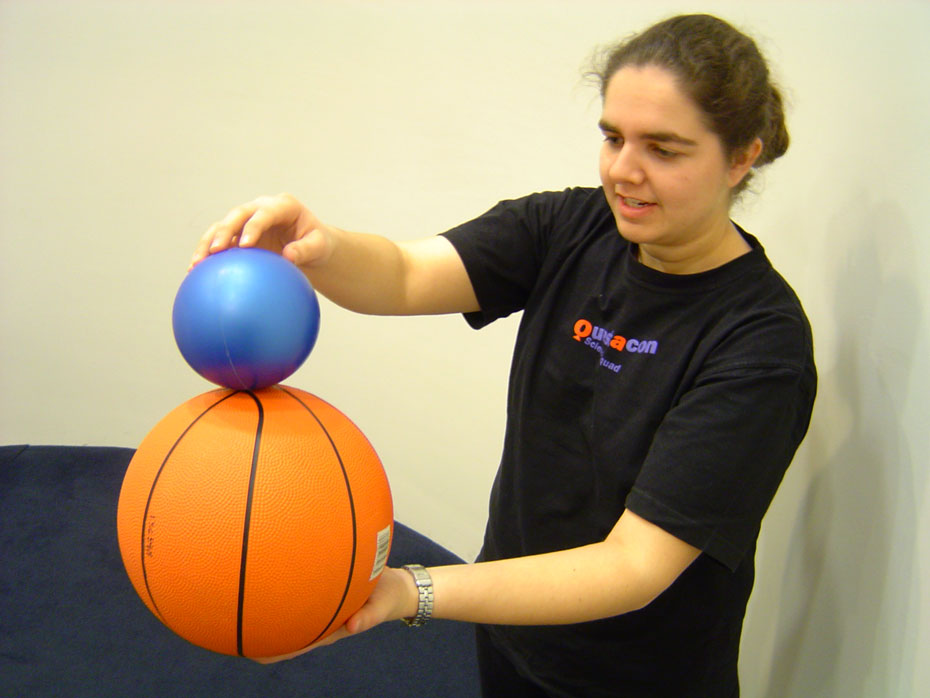more dates coming soon!# Bouncing With EnergyPractice those million dollar catches using two balls instead of one and watch one of the balls fly high into the sky!

• Science > Physical Sciences > Foundation > ACSSU005
• Science > Physical Sciences > Year 4 > ACSSU076
• Science > Physical Sciences > Year 8 > ACSSU155

## You'll need

• small inflated rubber ball (smaller and lighter than the basketball)

## Try this

1. Go outside and find an open space.
2. Hold the basketball in one hand and use your other hand to balance the small ball carefully on top of the basketball.
3. Gently hold the small ball in place and drop the two balls at exactly the same time. Observe what happens.
4. Repeat the activity with the balls reversed, so the basketball falls on top of the small ball. Observe what happens.

## What's happening?

When the basketball hits the ground, it quickly bounces back up and hits the small ball (which is still in the process of falling). As these two balls collide, the basketball transfers a lot of its energy to the smaller ball. As a result the small ball bounces very high.

This activity demonstrates how energy can be transferred from one object to another. When the two balls are held above the ground, they have stored or potential energy. When you let go of the balls, the potential energy changes into moving or kinetic energy, and they begin to fall to the ground. When the two balls collide, the kinetic energy is transferred from the basketball into the small ball, allowing the small ball to bounce high into the air.

This dramatic effect is due to the difference in masses between the two balls. The amount of kinetic energy an object has is linked to its mass and speed. If you want to get technical about things we can write out what's happening as a chemical equation:

Kinetic energy = ½ x mass x (velocity)2

This means that if both balls are moving at the same speed while they fall, the heavier ball will have more kinetic energy when the two balls hit the ground.
Most of the basketball's energy can be transferred to the small ball. Since the small ball has more kinetic energy than it had before, it must either gain mass or gain velocity so that the above equation remains true. As the small ball can't spontaneously obtain mass, it moves much faster instead and bounces high into the air. Since the basketball has transferred some of its kinetic energy to the small ball, it doesn't bounce as high as it normally would.

Swapping the positions of the balls demonstrates that the small ball doesn't have enough energy to transfer to the basketball because its mass is less than the basketball. As a result, the small ball is squashed under the weight of the heavier basketball.